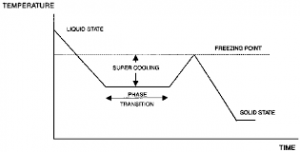## How to Calculate and Solve for Relative Freezing Time | Foundry TechnologyThe image above represents relative freezing time.

To compute for relative freezing time, four essential parameters are needed and these parameters are Constant (L), Constant (C), Volume of Riser/Volume of Casting (y) and Relative Contraction of Freezing (B).

The formula for calculating relative freezing time:

x = L / y – B + C

Where:

x = Relative Freezing Time
y = Volume of Riser / Volume of Casting
B = Relative Contraction on Freezing
C = Constant
L = Constant

Let’s solve an example;
Find the relative freezing time when the volume of riser/volume of casting is 26, the relative contraction on freezing is 21, the constant is 8 and the constant is 11.

This implies that;

y = Volume of Riser / Volume of Casting = 26
B = Relative Contraction on Freezing = 21
C = Constant = 11
L = Constant = 8

x = L / y – B + C
x = 8 / 26 – 21 + 11
x = 8 / 5 + 11
x = 1.6 + 11
x = 12.6

Therefore, the relative freezing time is 12.6 s.

Calculating the Constant (L) when the Relative Freezing Time, Constant (C), the Volume of riser/Volume of Casting and the Relative Contraction on Freezing is Given.

L = x (y – B) – C

Where;

L = Constant
x = Relative Freezing Time
y = Volume of riser / Volume of Casting
B = Relative Contraction on Freezing
C = Constant

Let’s solve an example;
Find the Constant when the relative freezing time is 24, the volume of riser / volume of casting is 14, the relative contraction on freezing is 8 and the constant is 10.

This implies that;

x = Relative Freezing Time = 24
y = Volume of riser / Volume of Casting = 14
B = Relative Contraction on Freezing = 8
C = Constant = 10

L = x (y -B) – C
L = 24 (14 – 8) – 10
L = 24 (6) – 10
L = 144 – 10
L = 134

Therefore, the constant is 134.